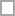# Expressions Quizzes & Trivia

A comprehensive database of expressions quizzes online, test your knowledge with expressions quiz questions. Our online expressions trivia quizzes can be adapted to suit your requirements for taking some of the top expressions quizzes.

Quiz Description

Questions: 26  |  Attempts: 689
• Sample Question
Which of the following does NOT mean multiplication?Questions: 9  |  Attempts: 64
• Sample Question
3x + 1 + 8x + 9Questions: 9  |  Attempts: 48
• Sample Question
How do you simplify: 5xyz + 9xyz - 2xyzQuestions: 5  |  Attempts: 47
• Sample Question
The following statement will result in a syntax error: double x = (96 + 84 + 99) / 3;This quiz will show your knowledge of evaluating expressions, writing expressions, and solving expressions with addition, subtraction, multiplication & division.

Questions: 20  |  Attempts: 32
• Sample Question
Evaluate 4m + 9 for m = 3.• Which of the following does NOT mean multiplication?Expressions question from

• The expression twice the product of 3 and x is the same as which of the following?Expressions question from

• The expression "four less than a number" is the same as which of the following?Expressions question from

• 3x + 1 + 8x + 9Expressions question from

• 2x + 5y - 7x + 8yExpressions question from

• 4(x + 8) - 9Expressions question from

• How do you simplify: 5xyz + 9xyz - 2xyzExpressions question from

• The following statement will result in a syntax error: double x = (96 + 84 + 99) / 3;Expressions question from

• The following statement will result in a syntax error: int n = 101 / 4;Expressions question from

• The following is a legal variable name:  4squareExpressions question from